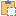#### Solid Class

A 3d solid.

Namespace: Autodesk.Revit.DB
Assembly: RevitAPI (in RevitAPI.dll) Version: 2015.0.0.0 (2015.0.0.0)

# Syntax

C#
``public class Solid : GeometryObject``
Visual Basic
``````Public Class Solid _
Inherits GeometryObject``````
Visual C++
``public ref class Solid : public GeometryObject``

# Remarks

A solid is defined by the faces and edges that form its boundary.

# ExamplesCopy C#
``````private void GetAndTransformSolidInfo(Application application, Element element, Options geoOptions)
{
// Get geometry element of the selected element
Autodesk.Revit.DB.GeometryElement geoElement = element.get_Geometry(geoOptions);

// Get geometry object
foreach (GeometryObject geoObject in geoElement)
{
// Get the geometry instance which contains the geometry information
Autodesk.Revit.DB.GeometryInstance instance = geoObject as Autodesk.Revit.DB.GeometryInstance;
if (null != instance)
{
foreach (GeometryObject instObj in instance.SymbolGeometry)
{
Solid solid = instObj as Solid;
if (null == solid || 0 == solid.Faces.Size || 0 == solid.Edges.Size)
{
continue;
}

Transform instTransform = instance.Transform;
// Get the faces and edges from solid, and transform the formed points
foreach (Face face in solid.Faces)
{
Mesh mesh = face.Triangulate();
foreach (XYZ ii in mesh.Vertices)
{
XYZ point = ii;
XYZ transformedPoint = instTransform.OfPoint(point);
}
}
foreach (Edge edge in solid.Edges)
{
foreach (XYZ ii in edge.Tessellate())
{
XYZ point = ii;
XYZ transformedPoint = instTransform.OfPoint(point);
}
}
}
}
}

}``````Copy VB.NET
``````Private Sub GetAndTransformSolidInfo(application As Application, element As Element, geoOptions As Options)
' Get geometry element of the selected element
Dim geoElement As Autodesk.Revit.DB.GeometryElement = element.Geometry(geoOptions)

' Get geometry object
For Each geoObject As GeometryObject In geoElement
' Get the geometry instance which contains the geometry information
Dim instance As Autodesk.Revit.DB.GeometryInstance = TryCast(geoObject, Autodesk.Revit.DB.GeometryInstance)
If instance IsNot Nothing Then
For Each instObj As GeometryObject In instance.SymbolGeometry
Dim solid As Solid = TryCast(instObj, Solid)
If solid Is Nothing OrElse 0 = solid.Faces.Size OrElse 0 = solid.Edges.Size Then
Continue For
End If

Dim instTransform As Transform = instance.Transform
' Get the faces and edges from solid, and transform the formed points
For Each face As Face In solid.Faces
Dim mesh As Mesh = face.Triangulate()
For Each ii As XYZ In mesh.Vertices
Dim point As XYZ = ii
Dim transformedPoint As XYZ = instTransform.OfPoint(point)
Next
Next
For Each edge As Edge In solid.Edges
For Each ii As XYZ In edge.Tessellate()
Dim point As XYZ = ii
Dim transformedPoint As XYZ = instTransform.OfPoint(point)
Next
Next
Next
End If
Next

End Sub``````